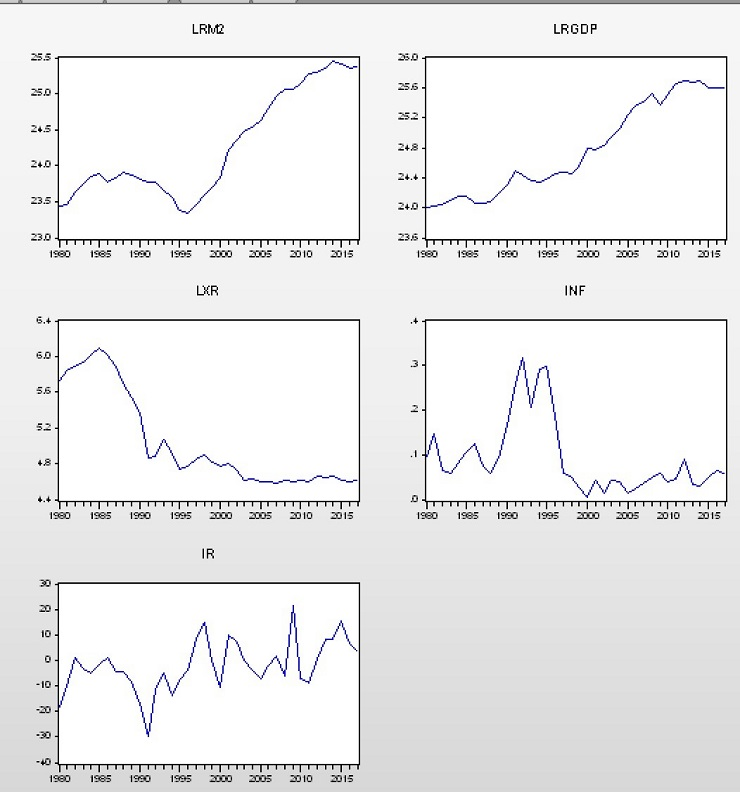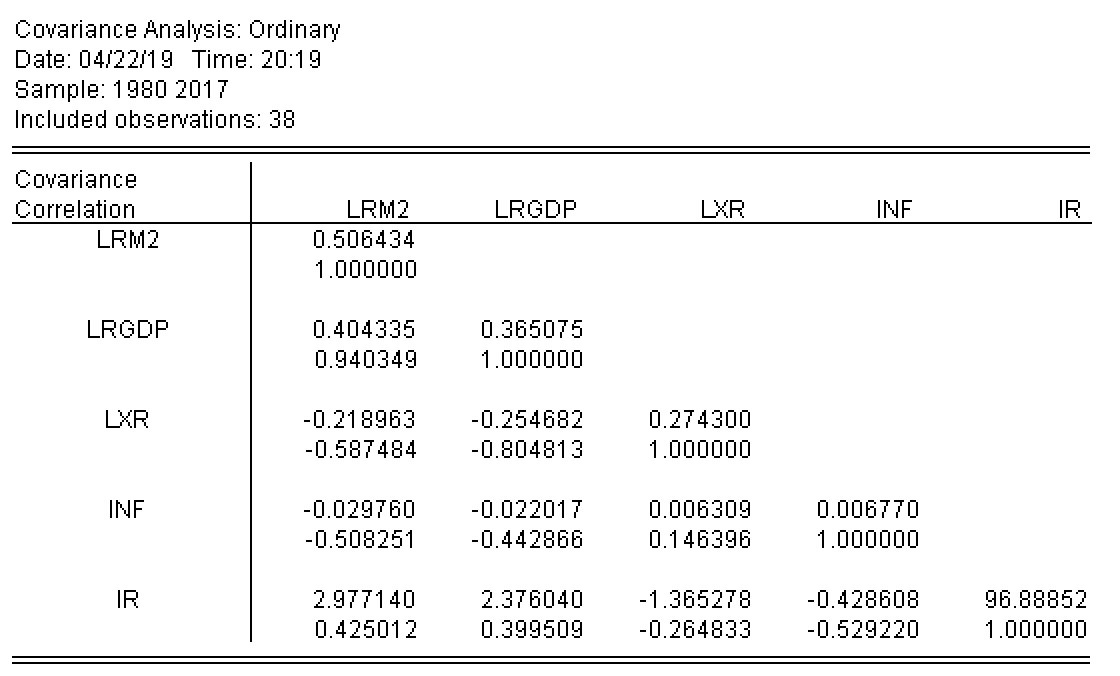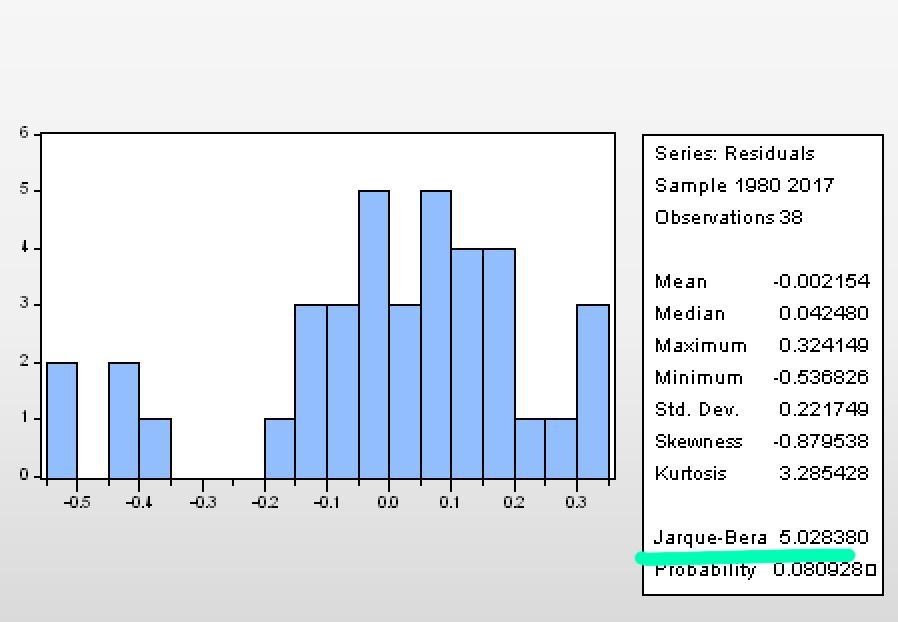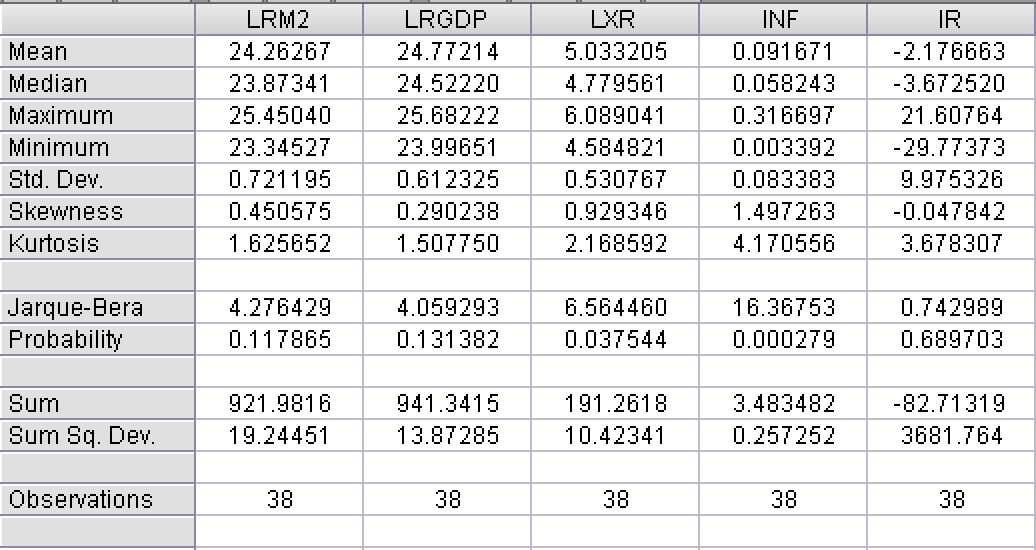# Money demand Sample Assignment

## Abstract

Money demand is an important aspect for any economy.

Money demand is said to be dependent on the RGDP, inflation, exchange rate and interest rate.

Data has been collected for Algeria country for all the four variables from 1980 to 2015.The trend of demand of money and RGDP is similar. The demand of money is represented by M2. The log of demand and RGDP is taken here in this model.

The demand of money decreased during the 1995 period but then it increased. The demand decreased from 1985 to 1995 slowly then it started increasing. At the same it is visible that the inflation rose for the economy drastically. As the inflation subsided the demand of money showed an increased.

The exchange rates for Algeria have been decreasing after increasing in the initial years. Then the rates decreased and it becomes flattish for the last few years. Inflation shows a lot variation however overall it decreased.

The interest rate for Algeria shows a lot of variation, in near 1985 to 1991 the interest rate decreased sharply showing a rate below -10%. However it increased from 1991 till 1998 to interest rate that is above negative nearly 15%.

RGDP also shows a uptrend. However, it has not been a complete uptrend. It shows three main uptrends.

## Correlation Analysis

Below table gives the correlation between the variables:Correlation between the variables shows demand of money is positively correlation with RGDP for Algeria. The value is 0.94 which indicates that there is strong positive correlation.

Correlation of demand of money with inflation and exchange rates is negative. This means that with these two variables move in opposite direction. This also is visible from the graph.

Correlation between the variables of demand of money and interest rate is 0.425 and it is positive correlation.## Descriptive Statistics

Descriptive statistics for all the variables is shown below:Mean of demand of money is 24.26, maximum value is 25.45 and minimum value is 23.3. There is not much of variation in demand of money.

Mean of log of RGDP is 24.77, maximum value is 25.68 and minimum value is 23.99.

Mean of LXR is 5.03 the maximum value is 6.08 and the minimum value is 4.58.

Mean of inflation is 0.09 and maximum value is 0.31 and minimum value is 0.003. Thus, inflation has shown a lot of variation.

Mean of IR is -2.17 and the maximum value is 21.6 and the minimum value is -29.7

We have used logarithm to (money, RGDP, XR). We got LM2 and RGDP by dividing them by consumer price index (CPI).